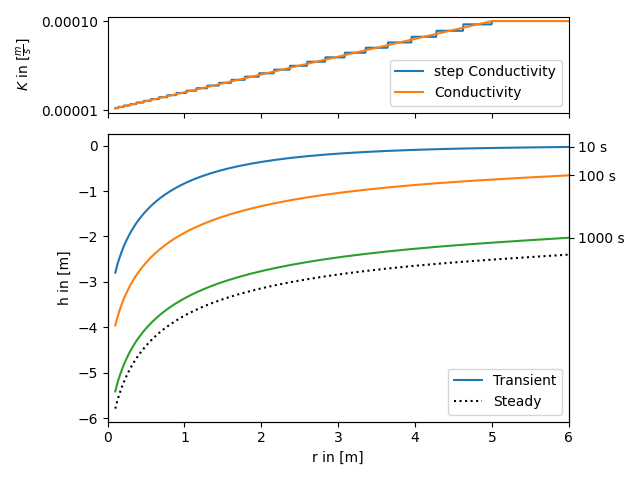# Self defined radial conductivity or transmissivity

All heterogeneous solutions of AnaFlow are derived by calculating an equivalent step function of a radial symmetric transmissivity resp. conductivity function.

The following code shows how to apply this workflow to a self defined transmissivity function. The function in use represents a linear transition from a local to a far field value of transmissivity within a given range.

The step function is calculated as the harmonic mean within given bounds, since the groundwater flow under a pumping condition is perpendicular to the different annular regions of transmissivity.

Reference: (not yet published)import matplotlib.gridspec as gridspec
import numpy as np
from matplotlib import pyplot as plt

from anaflow.tools import annular_hmean, specialrange_cut, step_f

"""Conductivity with linear increase from K_well to K_far."""
return np.minimum(np.abs(rad) / len_scale, 1.0) * (K_far - K_well) + K_well

time_labels = ["10 s", "100 s", "1000 s"]
time = [10, 100, 1000]

K_well = 1e-5
K_far = 1e-4
len_scale = 5.0
dim = 1.5

rate = -1e-4
S = 1e-4

cut_off = len_scale
parts = 30
r_well = 0.0
r_bound = 50.0

# calculate a disk-distribution of "trans" by calculating harmonic means
R_part = specialrange_cut(r_well, r_bound, parts, cut_off)
K_part = annular_hmean(
cond, R_part, ann_dim=dim, K_far=K_far, K_well=K_well, len_scale=len_scale
)
S_part = np.full_like(K_part, S)
r_bound,
cond,
dim=dim,
rate=-1e-4,
K_far=K_far,
K_well=K_well,
len_scale=len_scale,
)

# plotting
gs = gridspec.GridSpec(2, 1, height_ratios=[1, 3])
ax1 = plt.subplot(gs)
ax2 = plt.subplot(gs, sharex=ax1)
time_ticks = []
for i, step in enumerate(time):
label = "Transient" if i == 0 else None

ax1.plot(
)
ax1.set_yticks([K_well, K_far])
ax1.set_ylabel(r"$K$ in $[\frac{m}{s}]$")
plt.setp(ax1.get_xticklabels(), visible=False)
ax1.legend()
ax2.set_xlabel("r in [m]")
ax2.set_ylabel("h in [m]")
ax2.legend()